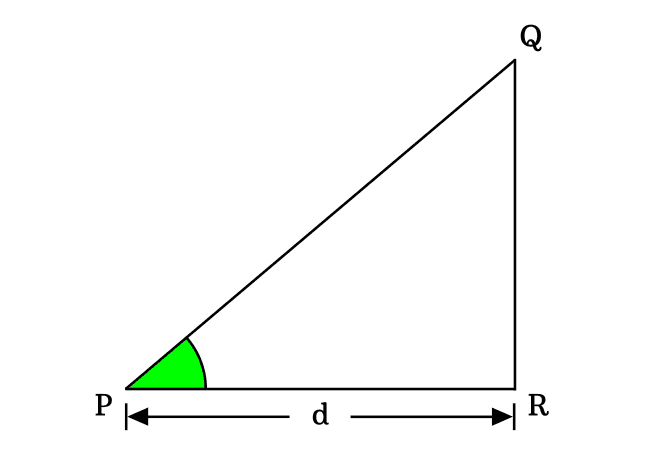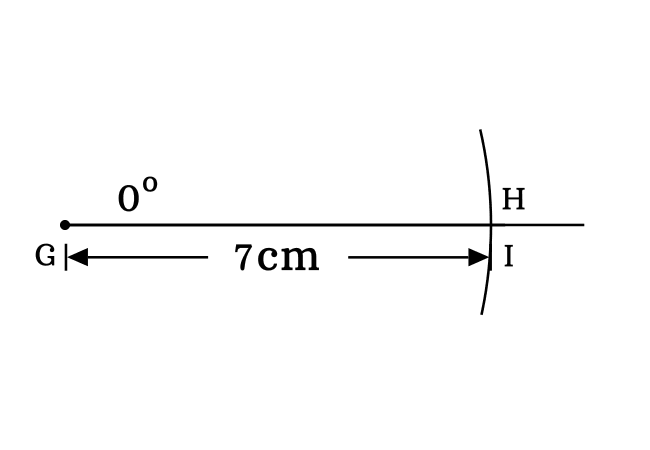# $\tan{(0^°)}$ Proof

According to trigonometry, the tan of zero degrees is equal to zero.

$\tan{(0^°)} \,=\, 0$

In mathematics, the tan of angle zero degrees can be derived in three different methods and let’s learn each mathematical approach for proving that the tan of angle zero degrees is equal to zero.

### Fundamental method

We consider a geometric property between sides of a triangle for deriving the exact value of tangent of angle zero degrees in this method. Therefore, let’s learn it by imagining a right triangle with zero degrees angle. The $\Delta QPR$ is an example for a zero angle right triangle.The tan function can be written in ratio form of lengths of the sides as per its fundamental definition. If the angle of a right triangle is zero degrees, then the tan function is written as $\tan{(0^°)}$.

$\implies$ $\tan{(0^°)}$ $\,=\,$ $\dfrac{Length \, of \, Opposite \, side}{Length \, of \, Adjacent \, side}$

$\implies$ $\tan{(0^°)}$ $\,=\,$ $\dfrac{QR}{PR}$

Actually, the length of opposite side is zero in a zero degree right triangle. Therefore $QR \,=\, 0$.

$\implies$ $\tan{(0^°)}$ $\,=\,$ $\dfrac{0}{d}$

$\,\,\,\therefore\,\,\,\,\,\,$ $\tan{(0^°)}$ $\,=\,$ $0$

### Experimental method

In this method, we use some geometric tools for proving the tangent of angle zero degrees practically by constructing a right triangle with zero degrees angle. Therefore, let’s begin the process of constructing a right angled triangle with zero degrees angle.1. From point $G$, draw a straight line in horizontal direction by a ruler.
2. Draw a zero degree line by coinciding the middle point with point $G$ and also coinciding its right side baseline with horizontal line. Actually, the zero degrees line is drawn over the horizontal line.
3. Later, set the distance between needle point and pencil lead point of a compass to any length (for example $7$ centimetres). After that, draw an arc on zero degrees straight line and it cuts the zero angle line at point $H$.
4. Finally, draw a perpendicular line to horizontal line from point $H$ by a ruler and it intersects the horizontal line at point $I$ for completing the construction of $\Delta HGI$. Remember that it is impossible to draw a perpendicular line to horizontal line from point $H$ because the positions of both points $H$ and $I$ are same in the plane.In $\Delta HGI$, the opposite side, adjacent side and hypotenuse are $\overline{HI}$, $\overline{GI}$ and $\overline{GH}$ respectively. $HI = 0\,cm$, $GI = 7\,cm$ and $GH = 7\,cm$. Now, calculate the tangent of angle zero degrees by the ratio of lengths of its respective sides.

$\tan{(0^°)} \,=\, \dfrac{HI}{GI}$

$\implies$ $\tan{(0^°)} \,=\, \dfrac{0}{7}$

$\,\,\,\therefore\,\,\,\,\,\,$ $\tan{(0^°)} \,=\, 0$

### Trigonometric method

The tangent of angle zero degrees can be proved in trigonometric mathematics by the fundamentals of trigonometry. According to quotient trigonometric identity, the tan function can be expressed in terms of sine and cos function.

$\tan{\theta} \,=\, \dfrac{\sin{\theta}}{\cos{\theta}}$

Here, the angle $\theta$ is zero degrees.

$\implies$ $\tan{(0^°)} \,=\, \dfrac{\sin{(0^°)}}{\cos{(0^°)}}$

Actually, the value of sine of zero degrees is equal to zero and the value of cos of zero degrees is one. Now, substitute them in the above equation for calculating the tan of $0$ degrees.

$\implies$ $\tan{(0^°)} \,=\, \dfrac{0}{1}$

$\,\,\,\therefore\,\,\,\,\,\,$ $\tan{(0^°)} \,=\, 0$

In this way, the tangent of angle zero degrees can be derived in mathematics in three different methods possibly.

Latest Math Topics
Jun 26, 2023
Jun 23, 2023

Latest Math Problems
Jul 01, 2023
Jun 25, 2023
###### Math Questions

The math problems with solutions to learn how to solve a problem.

Learn solutions

Practice now

###### Math Videos

The math videos tutorials with visual graphics to learn every concept.

Watch now

###### Subscribe us

Get the latest math updates from the Math Doubts by subscribing us.